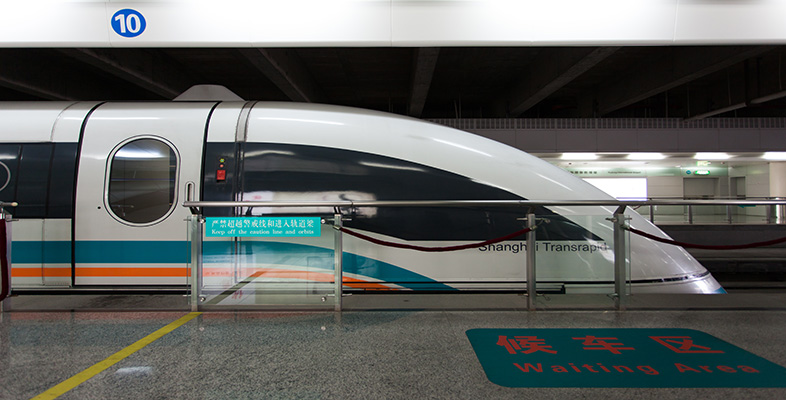Superconductivity

Start this free course now. Just create an account and sign in. Enrol and complete the course for a free statement of participation or digital badge if available.

# 2.2 Persistent currents lead to constant magnetic flux

An important consequence of the persistent currents that flow in materials with zero resistance is that the magnetic flux that passes through a continuous loop of such a material remains constant. To see how this comes about, consider a ring of metal, enclosing a fixed area A, as shown in Figure 6a. An initial magnetic field B﻿0 is applied perpendicular to the plane of the ring when the temperature is above the critical temperature of the material from which the ring is made. The magnetic flux Φ through the ring is B﻿0﻿A, and if the ring is cooled below its critical temperature while in this applied field, then the flux passing through it is unchanged. If we now change the applied field, then a current will be induced in the ring, and according to Lenz's law the direction of this current will be such that the magnetic flux it generates compensates for the flux change due to the change in the applied field. From Faraday's law, the induced emf in the ring is −﻿d﻿Φ﻿/﻿d﻿t = −﻿A﻿d(B − B﻿0)﻿/﻿d﻿t, and this generates an induced current I given by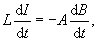where L is the self-inductance of the ring. Note that there is no ohmic term, IR, on the left-hand side of this equation, because we are assuming that R = 0. Integrating this equation, we obtain

LI + BA = constant.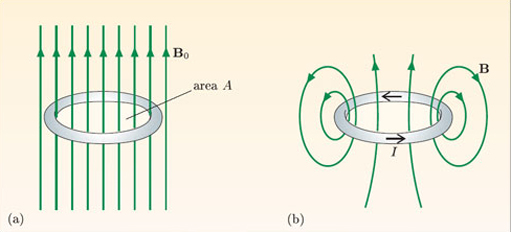Figure 6 (a) A ring cooled below its critical temperature in an applied field B0. (b) When the applied field is removed, a superconducting current maintains the flux through the ring at the same value.

But LI is the amount of flux passing through the ring generated by the current I flowing in the ring – this is just the definition of self-inductance L – so (﻿LI﻿ + BA) is the total magnetic flux through the ring. The total flux threading a circuit with zero resistance must therefore remain constant – it cannot change. If the applied magnetic field is changed, an induced current is set up that creates a flux to compensate exactly for the change in the flux from the applied magnetic field. Because the circuit has no resistance, the induced current can flow indefinitely, and the original amount of flux through the ring can be maintained indefinitely. This is true even if the external field is removed altogether; the flux through the ring is maintained by a persistent induced current, as in Figure 6b. However, note that constant flux through the ring does not mean that the magnetic field is unchanged. In Figure 6a there is a uniform field within the ring, whereas in Figure 6b the field is produced by a current flowing in the ring and will be much larger close to the ring than at its centre.

An important application of the constant flux through a superconducting circuit is shown in Figure 7. A superconducting solenoid, used to produce large magnetic fields, is connected to a power supply that can be adjusted to provide the appropriate current to generate the required field. For some applications it is important for the field to remain constant to a higher precision than the stability of the power supply would allow. A stable field is achieved by including a superconducting switch in parallel with the solenoid. This is not a mechanical switch, but a length of superconducting wire that is heated to above its critical temperature to ‘open’ the switch, and cooled below the critical temperature to ‘close’ it. With the switch open, the current from the power supply is set to give the required field strength. The switch is then closed to produce a completely superconducting circuit that includes the solenoid, the switch and the leads connecting them. The flux through this circuit must remain constant in time, so the field inside the solenoid will also remain constant in time. An added bonus is that the power supply can now be disconnected, which means that no energy is being dissipated while maintaining the field.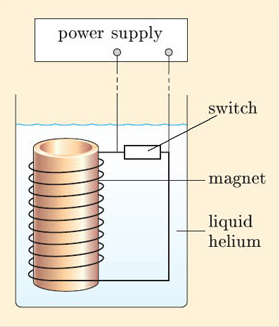Figure 7 A superconducting solenoid with a superconducting switch that allows it to operate in a persistent current mode.

Superconducting coils with persistent currents can be used in high-speed magnetically-levitated trains. In the system used on the Yamanashi Maglev Test Line in Japan (Figure 8), superconducting coils mounted on the sides of the train induce currents in coils mounted in the walls of a guideway, and the attractive and repulsive forces between the superconducting magnets and the track-mounted coils both levitate the train and provide lateral guidance. The train is propelled forwards by attractive and repulsive forces between the superconducting magnets and propulsion coils located on the walls of the guideway that are energised by a three-phase alternating current that creates a shifting magnetic field along the guideway. In 2003, a train reached the record-breaking speed of 581 km h﻿−﻿1 on this track.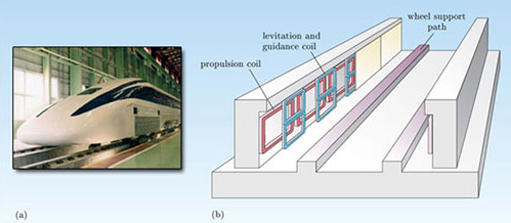Figure 8 (a) A train that uses superconducting coils for magnetic levitation. (b) The guideway for the train, showing the coils used for levitation, guidance and propulsion.

A second application is the use of a superconducting tube to screen sensitive components from magnetic fields, as shown in Figure 9. The tube is cooled below its critical temperature in a very small magnetic field. If a magnetic field is subsequently applied in the region of the tube, screening currents will be induced that generate fields which cancel out the applied field within the tube. However, note that effective screening requires a long tube, because only this geometry will generate a uniform magnetic field in the middle of the tube.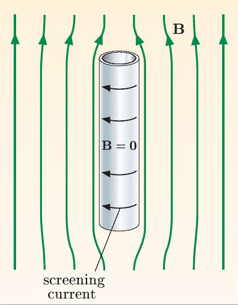Figure 9 A long superconducting tube screens the region inside from externally applied magnetic fields.